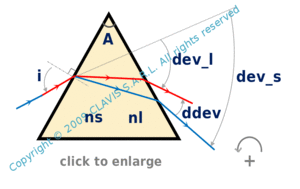and orgeometrical optics
basics

dispersion by a prism

Caution
Fill in all input cells.
Choose all parameters unit.
ns and nl can be either values or reference of a glass from the catalog. If values are directly entered, the wavelength will not be considered.
Except A which is positive, all angles are algebric.
Angle positive way is counterclockwise according the round arrow in the bottom right of the scheme.

InputValueunit
A
ns
nl
i
OutputValueUnit
Disto be calculated
Dilto be calculated
dDito be calculatedA : prism angle ns : refraction index corresponding to the smallest wavelength nl : refraction index corresponding to the largest wavelength Dis : total deviation for the smallest wavelength Dil : total deviation for the largest wavelength dDi : dispersion of deviation ( Dis - Dil )

Note
This calculation page can not detect all unrealistic inputs.